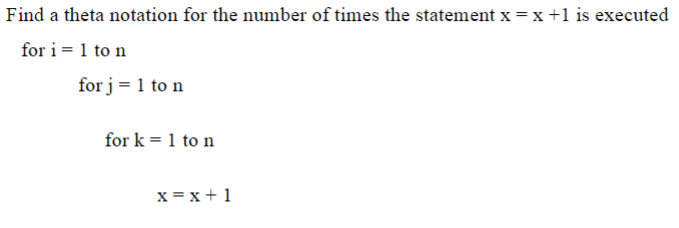# Find a theta notation for the number of times the statement x = x +1 is executedfor i = 1 to nfor j= 1 to nfor k = 1 to nx = x+ 1

Question
4 views

Find the theta notation for the number of times "x = x + 1" is executed.help_outlineImage TranscriptioncloseFind a theta notation for the number of times the statement x = x +1 is executed for i = 1 to n for j= 1 to n for k = 1 to n x = x+ 1 fullscreen
check_circle

Step 1

For each iteration of the outer loop we have n iteration of the middle loop and for each iteration of the middle loop we have n iteration of the inner most loop. Hence f...

### Want to see the full answer?

See Solution

#### Want to see this answer and more?

Solutions are written by subject experts who are available 24/7. Questions are typically answered within 1 hour.*

See Solution
*Response times may vary by subject and question.
Tagged in